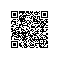# EM算法是炼金术吗？

EM算法的九层境界：Hinton和Jordan理解的EM算法

P(X|θj)=Kexp[-(X-cj)2/(2dj2)]，j=1，2，…，n

P(X)=P*(y1)P(X|θ*1)+P(y2)P(X|θ*2)

Q(X)=P(y1)P(X|θ1)+P(y2)P(X|θ2)

H(Q||P)=∑iP(xi)log[P(xi)/P(xi|θ)]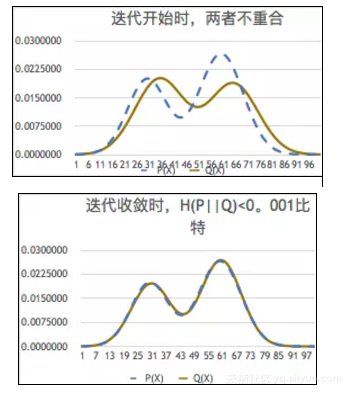EM算法起源于这篇文章：Dempster, A.P., Laird, N.M., Rubin,D. B.: Maximum Likelihood from Incomplete Datavia the EM Algorithm. Journal of the Royal Statistical Society, Series B39, 1–38(1977)。通过这个网站http://www.sciencedirect.com/搜索可见，光是标题有EM算法的英文文章就有6.8万篇(有似然度的文章将近76万篇)，可见研究和应用EM算法的人何其多。

Wiki百科上的EM算法介绍见这里：

https://en.wikipedia.org/wiki/Expectation%E2%80%93maximization_algorithm

http://www.cnblogs.com/mindpuzzle/archive/2013/04/05/2998746.html

EM算法的基本思想是：

Hθ’(X)=∑iP(xi)logP(xi|θ)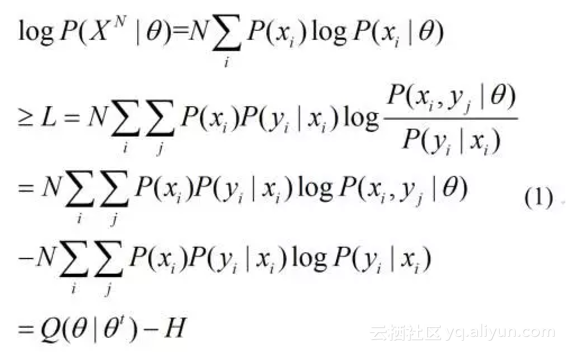[Q(θ|θt)-H]/N=Hθ’(X，Y)-Hθ’(Y|X)=-Hθ(X，Y)+Hθ(Y|X)

EM算法分两步：

E-step：写出预测的yj的条件概率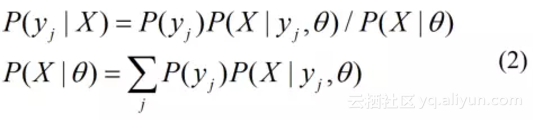M-step：最大化Q(θ|θt)，也就是最大化负的联合熵Hθ’(XY)，或最小化联合交叉熵Hθ(XY)。

1. Expectation-maximization works to improve Q(θ|θt) rather than directly improving log(X|θ). Here is shown that improvements to the former imply improvements to the latter.

2）M-step可以增大Q(.)，E-step也不减少Q(.)，所以反复迭代就能收敛。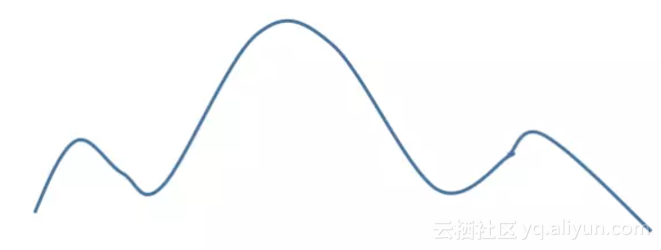EM有时虽然能收敛，但是常常收敛很慢。为了提高速度，于是又有很多人提出很多改进办法。其中比较出名的一个就是上面《九层境界》中提到的VBEM算法(详见Neal, R.，Hinton, G.: A view of the EM algorithm that justifies incremental, sparse, and other variants. in: Michael I. Jordan(ed.) Learning in Graphical Models，pp355–368. MITPress，Cambridge，MA(1999))，就是用

F(θ，q)=Q(θ|θt)+H(Y)

H(X，Y)=H(X)+H(Y|X)

Hθ(X，Y)=Hθ(X)+Hθ(Y|X)=-logP(X|θ)+Hθ(Y|X)

H’θ(X，Y)=logP(X|θ)+H’θ(Y|X)

H’θ(X，Y)+H(Y)=logP(X|θ)+H’θ(Y|X)+H(Y)
H(Y)-Hθ(X，Y)=-Hθ(X)+H(Y)-Hθ(Y|X)

-Hθ(X|Y)=-Hθ(X)+Iθ(X;Y

F(θ，q)=-Hθ(X|Y)=-Hθ(X)+Iθ(X;Y)(3)

F(θ，q)+H(X)=H(X)-Hθ(X|Y)=I(X;ϴ)(4)

H(Q||P)=H(X|θ)-H(X)=Iθ(X;Y)-I(X;ϴ)(5)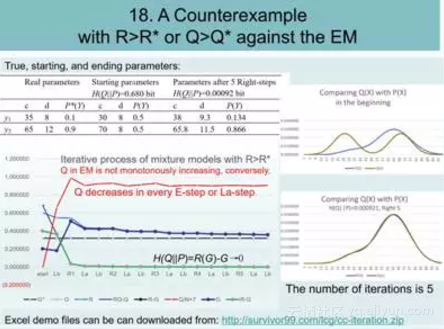F(.)有类似情况，它和预测互信息(图示是G)走势完全一致，在每个E步是下降的。原来影响交叉熵有三个因素：

1)预测的分布和样本的分布是否符合，如果更符合，负熵Q(.)和F(.)就更大；

2)X和Y的分布是否集中，如果更集中负熵就更大；

3)X和Y是否相关，如果更相关负熵就更大。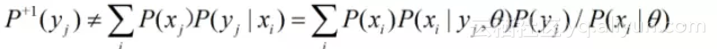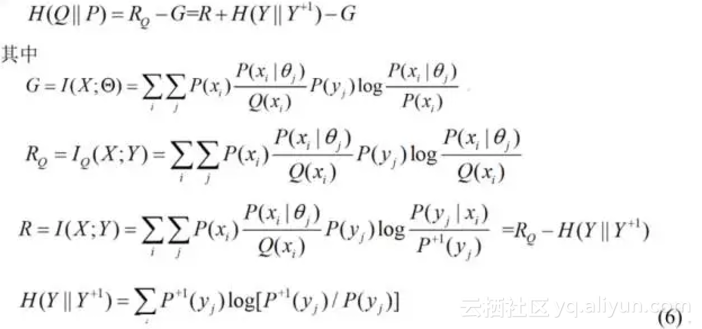I：写出Shannon信道表达式，等于EM算法中的E步;

II：调整P(Y)，使得P(Y+1)=P(Y);如果H(Q||P)<0.001，结束。

III:改变参数最大化语义互信息G。转到I。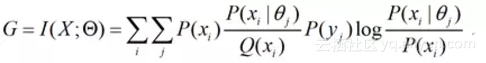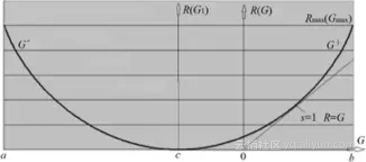《从“炼金术”到“电力”的机器学习》，他并没有否定深度学习，只是说要加强理论研究，也不排除先有实践再有理论。